# Electronics and Communication Engineering - Networks Analysis and Synthesis

1.

Which one is correct statement?

1. Circuit is always open
2. Network is always open
3. Circuit is always closed
4. Network is always closed

 A. 1, 2 B. 2, 3 C. 3, 4 D. 1, 4

Explanation:

No answer description available for this question. Let us discuss.

2.

The Q factor of a series RLC circuit is

 A.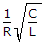B.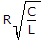C.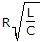D.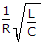Explanation:

No answer description available for this question. Let us discuss.

3.

The function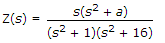A. is a reactance function B. is an RC function C. is an RL function D. may be reactance function or RC function

Explanation:

No answer description available for this question. Let us discuss.

4.

If the network in figure (1), (2) are equivalent at terminals A - B, then voltage V and resistance R are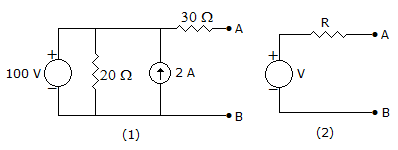A. 100V and 12 Ω B. 60V and 12 Ω C. 100V and 30 Ω D. 60V and 30 Ω

Explanation:

No answer description available for this question. Let us discuss.

5.

A source is delivering maximum power to a resistance through a network. The ratio of power delivered to the source power,

 A. is always 0.5 B. may be 0.54 or less C. may be 0.5 or less D. may be 0.5 or more